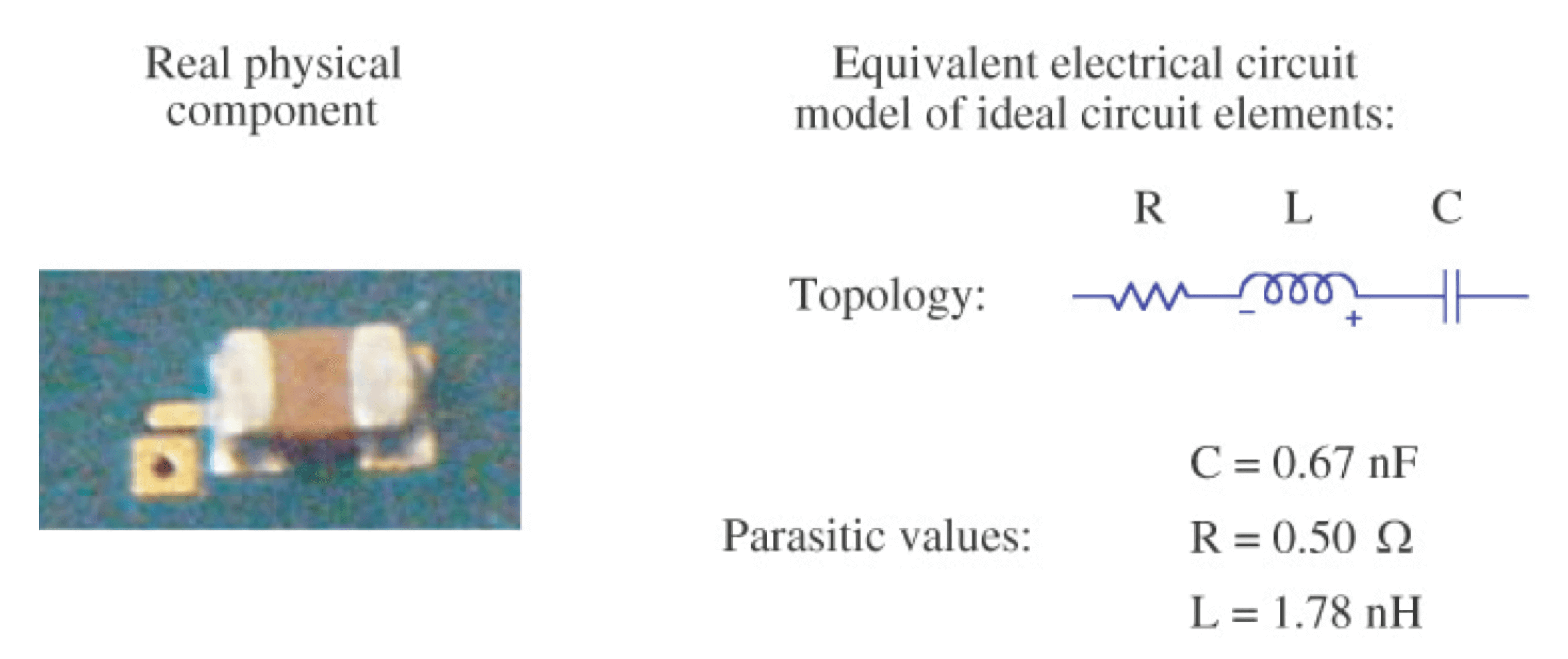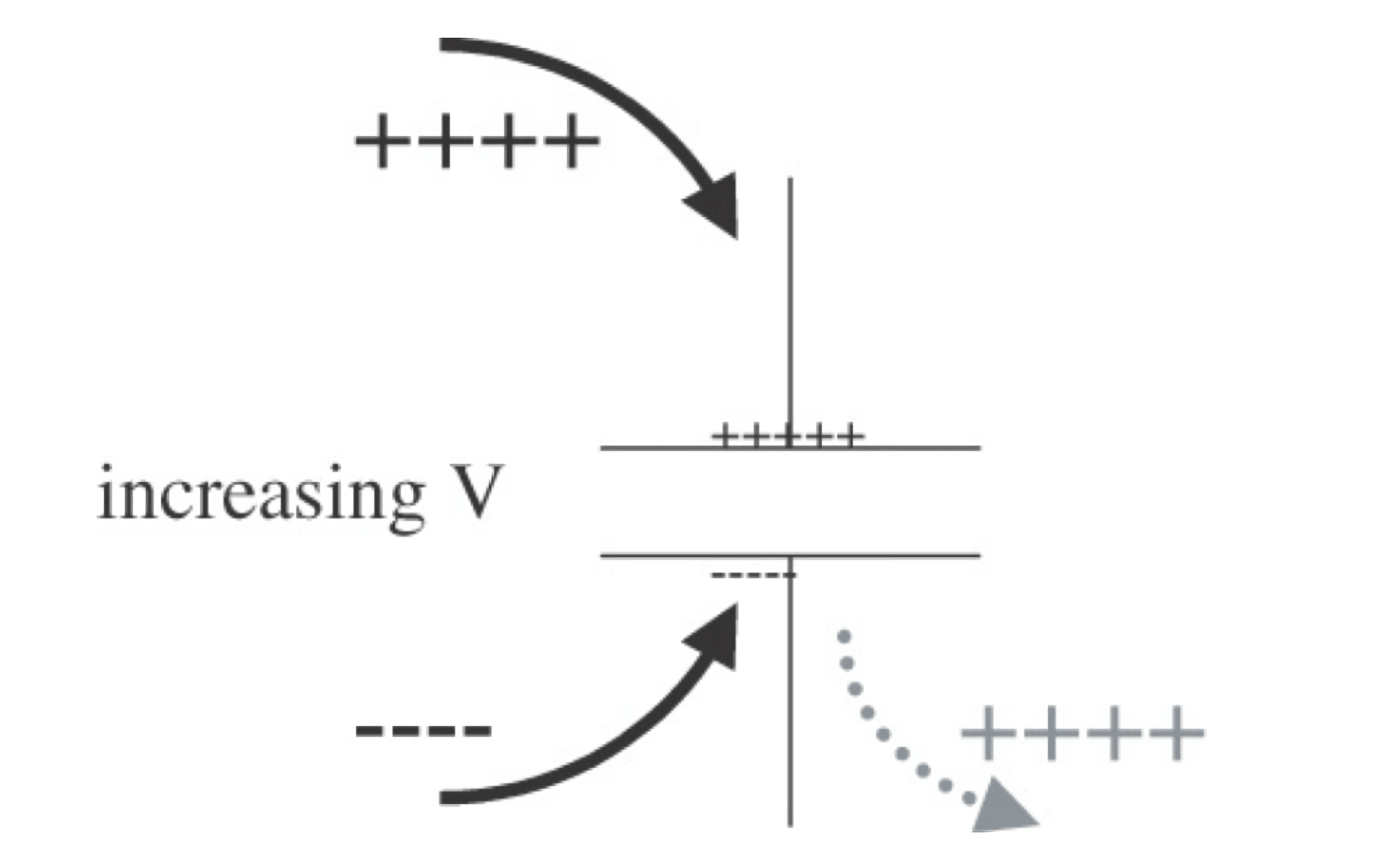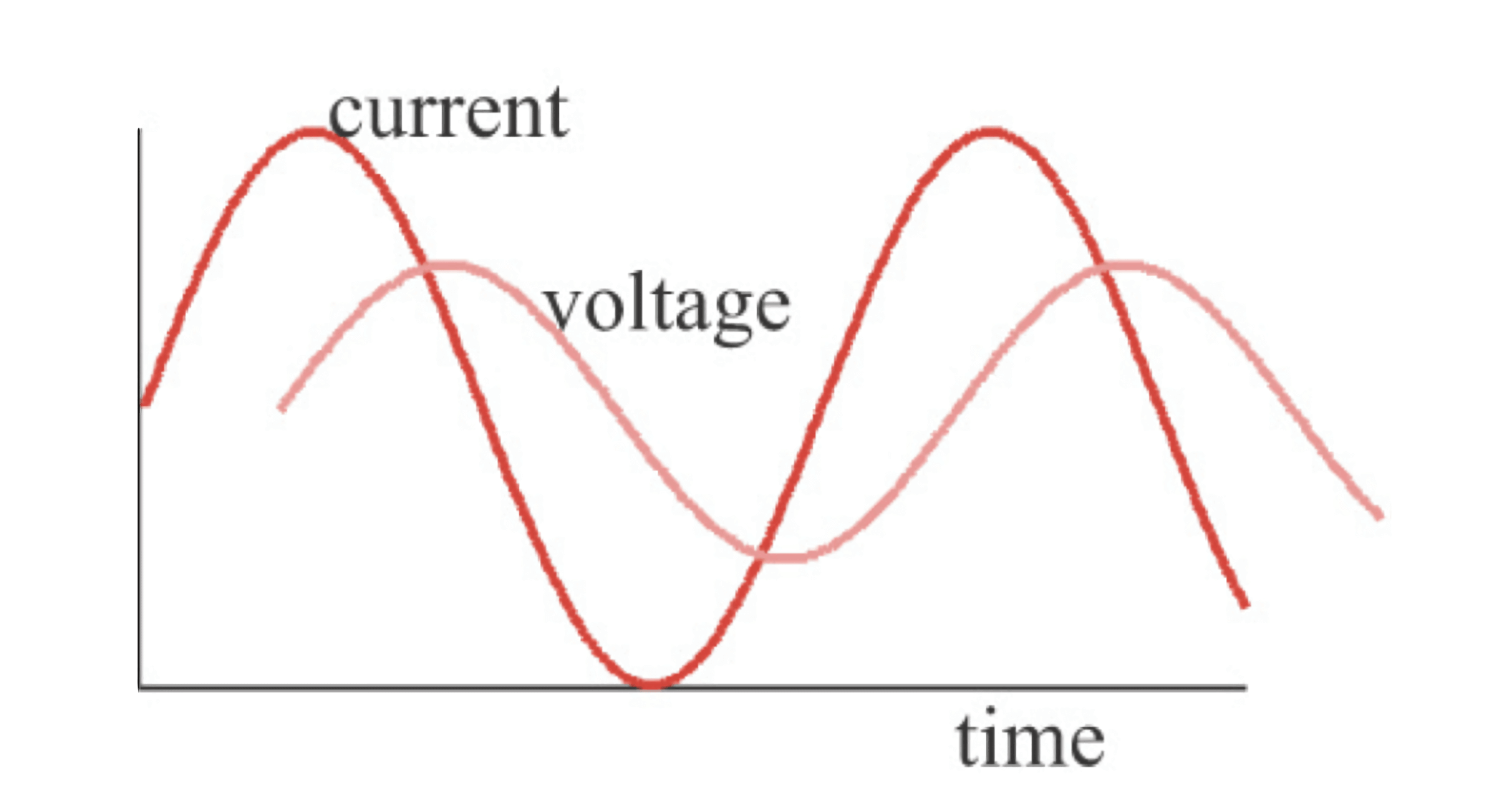# 信号完整性 - 阻抗与电气模型

## 基本电气模型

1. 理想电阻器
2. 理想电容器
3. 理想电感器
4. 理想传输线## 时域中理想电容器的阻抗

$C=\frac{Q}{V}$$I=\frac{dQ}{dt}=C\frac{dV}{dt}$

$Z=\frac{V}{I}=\frac{V}{C\frac{dV}{dt}}$

## 时域中理想电感器的阻抗

$V=L\frac{dI}{dt}$

$Z=\frac{V}{I}=L\frac{\frac{dI}{dt}}{I}$

## 频域中的阻抗

$\omega=2\pi f$$|Z|=\frac{|V|}{|I|}$

## 频域中理想电阻器的阻抗

$V=I_0 sin(\omega t) \cdot R$

$Z=\frac{V}{I}=\frac{I_0 sin(\omega t) \cdot R}{I_0 sin(\omega t)}=R$

## 频域中理想电容器的阻抗

$I=C\frac{d V_0sin(\omega t)}{dt}=C\omega V_0 cos(\omega t)$

$Z=\frac{V}{I}=\frac{V_0 sin(\omega t)}{C\omega V_0 cos(\omega t)}=\frac{1}{\omega C}\cdot \frac{sin(\omega t)}{cos(\omega t)}$

## 频域中理想电感器的阻抗

$I=L\frac{d I_0sin(\omega t)}{dt}=L\omega I_0 cos(\omega t)$

$Z=\frac{V}{I}=\frac{L\omega I_0 cos(\omega t)}{\omega I_0 sin(\omega t)}=\omega L \cdot \frac{cos(\omega t)}{sin(\omega t)}$

## 参考与致谢

• 《信号完整性与电源完整性分析》